Published: 15 November 2016

# Milling cutter condition reliability prediction based on state space model

Hongkun Li1
Shuai Zhou2
Honglong Kan3
Ming Cong4
1, 2, 3, 4School of Mechanical Engineering, Dalian University of Technology, Dalian, P. R. China
Corresponding Author:
Hongkun Li
Views 95

#### Abstract

Reliability analysis based on equipment's performance degradation characteristics is one of important research areas for reliability engineering. Many researcher work on multi-sample analysis, but it is limited for single equipment or small sample reliability prediction. Therefore, the method of reliability prediction based on state space model (SSM) is investigated in this research for small sample analysis. Firstly, signals about machine working conditions are collected based on-line monitoring technology. Secondly, wavelet packet energy parameters are determined based on the monitored signals. Frequency band energy is regarded as characteristic parameter. Then, the degradation characteristics of signal to noise ratio is improved by moving average filtering processing. In the end, SSM is established to predict degradation characteristics of probability density distribution, and the degree of reliability is determined. Milling cutter is used to demonstrate the rationality and effectiveness of this method. It can be concluded that this method is effective for milling cutter reliability estimation based on the data analysis. It also contributes to machine condition remaining useful life prediction.

## 1. Introduction

Reliability analysis based on equipment's performance degradation characteristics is one of the important research areas for machine safety operation. The operation equipment reliability estimation can let the operator accurately know equipment status, which is important to the safety production. The traditional methods about reliability analysis rely on large sample experiment and statistical approach. It is time-consuming and it can’t run for a small sample equipment, such as gas turbine, centrifugal compressor, and so on. In the most cases, there is no two same machines with identical condition. At the same time, the data is very changeable and difficult to collect. Therefore, the traditional analysis method is not suitable for prediction. How to effectively improve the small samples reliability estimation method is urgently needed, especially for single equipment prediction. Milling cutter (MC) is the key component during production process, such as centrifugal impeller, diesel engine cylinder head, and so on. It is important and keep in working in normal condition and know the reliability of the milling cutter. In the most cases, degradation is the main failure for MC.

Reliability reflects the health degree of equipment and provides important information for equipment remaining life. There are many researchers investigating on reliability analysis in this area. Chinnam et al. put the thrust and torque on-line monitored in the process of high-speed steel drill for running status analysis. Polynomial regression models including Gaussian white noise are investigated. Neural network is applied on life prediction process . LU et al. uses quadratic exponential smoothing time series forecasting model for real-time reliability estimation . ZHANG et al.  extract the characteristic indicators of vibration signals in the bearing life test-rig, such as RMS value, peak-peak value, and kurtosis. Combined with bearing temperature, there are in total four indicators. The recursive Bayesian analysis method is used to estimate the reliability. Gebraeelet al.  extract feature from the vibration signal of real-time monitoring bearing as degradation indicators. Neural network is used to predict life distribution of bearing. FONGpoints out that degradation situation of each individual equipment is unique because of the difference of operation environment and using conditions. Condition monitoring information in running can be made reliability evaluation and prediction effectively for single equipment . Cheng  conducts condition monitoring data to estimate reliability on individual equipment, the lack of experience information and many uncertainty in equipment operation are considered. Failure threshold and degradation model are difficult to be directly confirmed. Therefore, he proposes a reliability prediction method by adaptive failure threshold. This method is applied to bearing and high pressure cleaning pump successfully. Lin et al. demonstrates a procedure to extract useful condition indicators from vibration signals and use the proportional hazards model (PHM) to develop optimal maintenance policies for the gearboxes. Lin and Tseng  combine the Weibull PHMon vibration-based machine condition monitoring techniques to estimate several machine reliability statistics. All above mentioned methods are helpful to the development of reliability estimation. However, these methods require a specific mechanical knowledge and make many assumptions about condition parameters degradation paths.

Heng  presents a prognostics approach using feed forward neural network on pump vibration data. The model incorporated population characteristics and suspended condition trending data of historical units into prognostics. Ding  extracts the degradation characteristics from monitored bearing vibration signals and sets up two parameters Weibull Proportional Hazards Model combined with the state information of bearing. The reliability of railway locomotive bearing is evaluated by this method. Chen  extracts the relevant characteristic parameters from vibration signals of the tool cutting process combined with the tool condition, evaluates reliability and predicts the residual life of tools using Logistic regression model. The two methods need certain historical failure data. But historical failure data for some large equipments are usually difficult to get. He  proposes a variety of reliability evaluation methods based on mechanical equipment state information. He comes up with a reliability assessment and life prediction of bearing based on signal processing and feature extraction. These studies show that the degradation characteristic extracted from monitored equipment signals can accurately reflect the dynamic characteristic of the equipment performance degradation. It is reasonable and effective for reliability evaluation, and it makes up for the deficiency of the traditional reliability evaluation.

State space model (SSM) is a special form of Hidden Markov Model. It can be used to describe the dynamic process changing in time. In recent years, it is used on fault diagnosis and prediction of mechanical equipment by many scholars. Orchard  uses SSM for equipment diagnosis and forecast online. Remaining life distribution of planetary gear fault instance combined with particle filtering technology is used to validate the rationality of the model. GASPERIN extracts the degradation characteristics of gearbox and establishes the SSM of degradation characteristics to predict the remaining service life of gear box combined with the Monte Carlo simulation . SSM can describe the system state using the current and past minimum information form without needing a lot of historical data. For single equipment reliability, it is difficult to be evaluated using traditional reliability method. So this paper proposes a reliability prediction method based on SSM. This method does not rely on historical failure data, and can realize the real-time prediction of single equipment reliability. Kalman filter has good performance on on-line prediction. But it is not suitable for nonlinear time series estimation. It is difficult for the real monitored data analysis. Therefore, accurate feature extraction and improved Kalman filter is used in this research.

In this research, a new method for operational condition reliability estimation is developed by combining SSM and feature extraction. Wavelet packet feature extraction is used to determine the related parameter for reliability analysis. To improve the accuracy of reliability estimation, moving average is used for the extracted data. A MC operational condition estimation is carried out to verify the effectiveness of this method. The results show that this method can estimate to the operational condition estimation for small sample, which has good accuracy for reliability estimation. At the same time, it is simple and convenient for practical application. This paper is structured as follows. Section 2 presents condition monitoring and feature extraction for MC operational progress. Section 3 introduces theory of this state space model. Section 4 provides the data analysis by using this method for MC. Concluding remarks are given in Section 5.

## 2. Condition monitoring and feature extraction

### 2.1. Condition monitoring

Condition monitoring is of great importance in order to estimate the tool wear. Cutting force is used on condition estimation as it is directly related to wearing process. Our research aims to retrieve sufficient monitoring information of MC wear condition. It is in favour of cost reduction, implementation feasibility, and so on. As well, it is also not easy to implement the on-the-fly measurement of wearing capacity of MC in the actual production. Milling force, vibration signal, acoustic emission (AE) signal have been investigated used on MC wearing process. At present, the cutting force measurement is only used in the experimental study. But it is hard to be applied to the actual production for MC condition monitoring. In this research, AE signal is investigated on MC condition monitoring and fault diagnosis.

Milling test is conducted on the DONG YU JINGJI CMV- 850A machining center in Mold Center, Dalian University of Technology and the material is FV520B. FV520B is a special material for impeller production. Its high hardness keeps blade working in the designed condition. Choose domestic APOLL550 portable industrial PC and AEwin data acquisition software of PAC company for the acoustic emission signal data acquisition. The MC life experiment is carried in accelerated manner. The spindle speed is 1000 rpm, the cutting depth is 0.4 mm, and the feed rate is 400 mm/min. The acoustic emission signal sampling length is 512000 points and the sampling frequency ${F}_{s}$ is 2048 kHz. Samples are collected every 10 seconds and 240 sets of acoustic emission signals are collected before the cutter tool coming to the stage of failure. Fig. 1 presents the picture for the test-rig. In the experiment, cutting force and AE signal are monitored to determine the parameter for condition classification. Fig. 2 gives the picture for the comparison of MC in different working conditions. Which mean normal and wearing condition, respectively. Cutting force and AE are determined based on the experiment for different working conditions. Fig. 3 and Fig. 4 show the comparison for cutting force with time domain and frequency spectrum in different conditions. It is obvious with cutting force amplitude increase with wearing conditions. There is much difference between normal and serious wearing condition. It means that cutting force is highly related with wearing process. But it is not convenient to monitor in practical condition. AE signals are also obtained during the experiment. Fig. 5 and Fig.6 show the comparison for AE with time domain and frequency spectrum in different conditions. It can also determine that AE signal will increase with MC wearing process. Therefore, it can be used to demonstrate the wearing process. But further investigation should be carried on as there is much noise interference. Effectively feature extraction can improve the accuracy of pattern recognition. Therefore, characteristic parameters are investigated to determine the best one to demonstrate the wearing process.

Fig. 1Test rig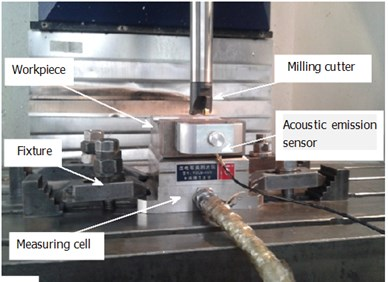Fig. 2Picture for cutting tools in different conditions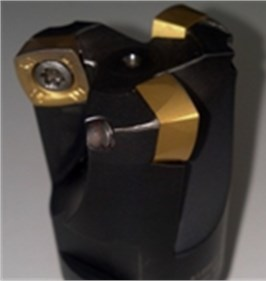a) Intact condition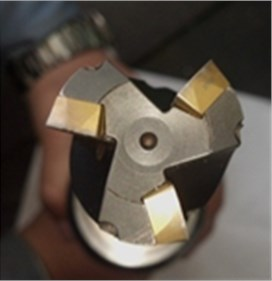b) Wear condition

Fig. 3Signal analysis for cutting force in normal condition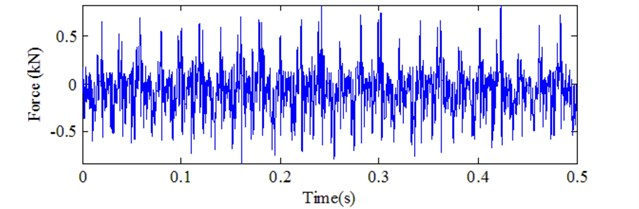a) Time domainb) Frequency spectrum

### 2.2. Feature extraction

Root mean square (RMS) has been broadly used on machine condition estimation and reliability prediction. But it is not sensitive to some signals and further extraction is urgently needed. Many researchers have worked on the signal processing technology. Vibration signal processing and feature extraction have been intensively investigated in the last two decades, such as time domain analysis, frequency domain analysis, time-frequency domain analysis. With the development of the wavelet analysis in last three decades, it has been broadly used to process vibration signals and the effect is remarkable . As the extension and development of wavelet transform, Wavelet Packet Decomposition (WPD) is multi-dimension process. Therefore, it has been investigated by many researchers.

Suppose there is a scaling space of limited energy signal ${U}_{0}^{0}$. Through wavelet packet transformation , ${U}_{0}^{0}$ is decomposed into several spaces in binary format. The iterative formula is:

1
${U}_{j+1}^{k}={U}_{j}^{2k}\oplus {U}_{k}^{2k+1},j\in Z,k\in {Z}^{+}.$

Fig. 4Signal analysis for cutting force in seriously wearing condition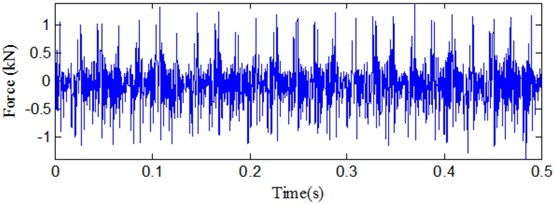a) Time domain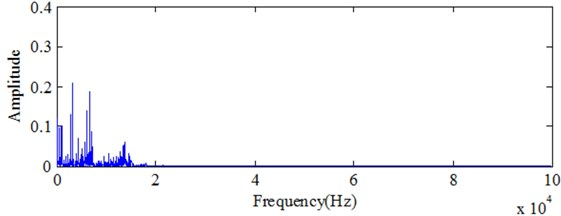b) Frequency spectrum

Fig. 5Signal analysis for AE in normal condition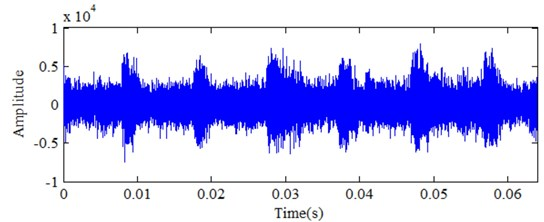a) Time domain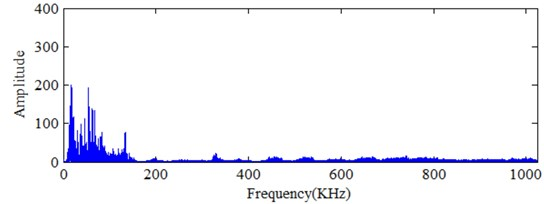b) Frequency spectrum

In Eq. (1), $j$$\left(j\le 0\right)$ is decomposition level, $\oplus$ expresses orthogonal decomposition, ${U}_{j+1}^{k}$, ${U}_{j}^{2k}$, and ${U}_{k}^{2k+1}$ three closure spaces respectively corresponding wavelet function are:

2
${\psi }_{2n}\left(t\right)=\sqrt{2}\sum _{kϵZ}h\left(k\right){\psi }_{n}\left(2t-k\right),$
3
${\psi }_{2n+1}\left(t\right)=\sqrt{2}\sum _{kϵZ}g\left(k\right){\psi }_{n}\left(2t-k\right).$

When $n=0$, ${\psi }_{0}\left(t\right)$ is a scaling function $\phi \left(t\right)$ and ${\psi }_{1}\left(t\right)$is a wavelet basis function $\psi \left(t\right)$, $h\left(k\right)$ and $g\left(k\right)$ are discrete quadrature mirror filter coefficients. The signals in the space ${U}_{j}^{n-1}$ can be obtained by wavelet packet function ${\psi }_{k}^{j,n}\left(t\right)$ reconstruction, which is shown in Eq. (4):

4
${s}_{j}^{n}\left(t\right)=\sum _{k\in Z}{D}_{k}^{j,k}{\psi }_{k}^{j,n}\left(t\right),k\in Z.$

Fig. 6Signal analysis for AE signal in seriously wearing condition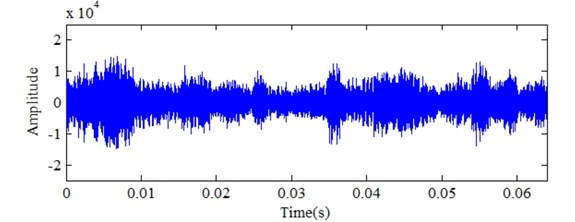a) Time domain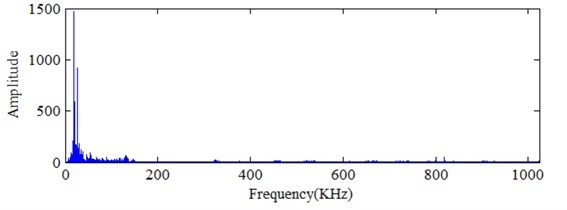b) Frequency spectrum

In Eq. (4), ${D}_{k}^{j,n}$ is wavelet packet coefficient, and it can be obtained by the following equation:

5
${D}_{k}^{j,k}={\int }_{-\infty }^{+\infty }f\left(t\right){\psi }_{k}^{j,n}\left(t\right)dt.$

The wavelet packet function ${\psi }_{j,k}\left(t\right)$ is orthogonal basis function in ${L}^{2}\left(R\right)$, so the energy of ${s}_{j}^{n}\left(t\right)$ is:

6
${WPE}_{n}=\sum _{k}{|{D}_{k}^{j,n}|}^{2}.$

The normalized wavelet packet energy is:

7
${E}_{n}=\frac{{WPE}_{n}}{\sum {WPE}_{n}}.$

### 2.3. Correlation analysis for AE and force

Zhong  did the correlation analysis on the related characteristics of acoustic emission signals and force signals with tool wear. The results show that acoustic emission characteristic is the same as force signal characteristic and it is highly correlated with tool wear. Fig. 10 provides different frequency energy bands distribution comparison at different conditions. The wearing condition is obvious larger than normal condition in the second frequency band. It also means that the second frequency band energy increases with MC degradation process. Fig. 7 gives the development trend for the wearing degradation with the second frequency band energy and cutting force. It is also the same result with Fig. 8. It can demonstrate cutting force be highly associated with the second frequency energy distribution. At the same time, the cutting force is also related with the wearing condition process. Thus, the relationship is analyzed by Fig. 9. It is almost the linear relationship for the cutting force and the second frequency band. Therefore, the second frequency band energy distribution can replace the cutting force to characterize the wearing process for reliability estimation.

Fig. 7Energy distribution comparison for different working conditions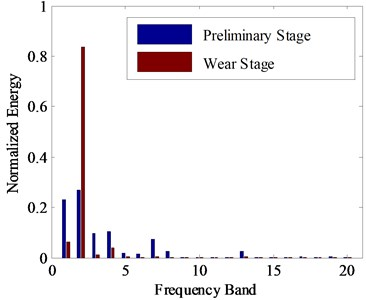Fig. 8Comparison for different trend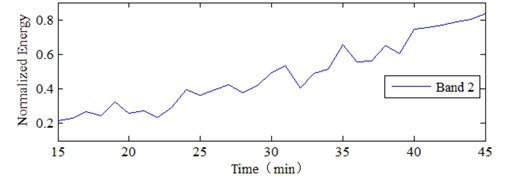a) Energy trend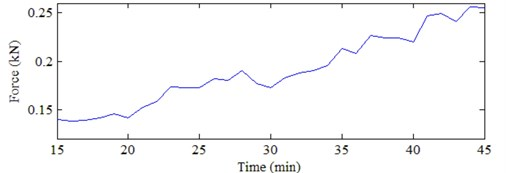b) Force trend

Fig. 9Relationship for Band 2 energy and cutting force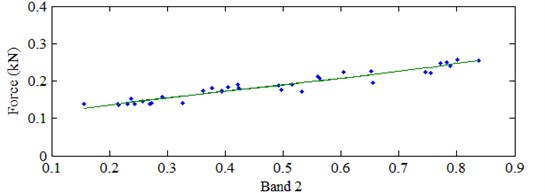## 3. State space model

### 3.1. Instantaneous reliability

Reliability is an important quality index of mechanical equipment, and the degree of reliability is one of the most important reliability evaluation indexes. The definition of reliability is that under prescribed conditions and within the limited time, the probability of equipment completes the required function without failure. However, in actual production the reliability based on probability statistics solution can’t help much. For analyzing and evaluating dynamic performance of the individual equipment, LU  firstly suggests the method to compute the reliability of equipment using interval integral defined by the failure threshold and index observation to predict the probability distribution of equipment performance degradation index. XU  uses the similar concept of reliability prediction and evaluation method and apply it in the instance estimation. If the instantaneous reliability of equipment at time $t$ is the probability which the device performance characteristics index ${y}_{t}$ is less than the failure threshold ${y}_{c}$:

8
$R\left(t\right)=P\left({y}_{t}\le {y}_{c}\right)={\int }_{0}^{{y}_{c}}{f}_{t}\left({y}_{t}\right)d{y}_{t}.$

In Eq. (8), ${f}_{t}\left({y}_{t}\right)$ is the probability density function of the state characteristic ${y}_{t}$. As shown in Fig. 13, the red line is the failure threshold set, the probability which the state characteristic is greater than the failure threshold.

### 3.2. State space model

Estimation method is important for accurate analysis. The degradation process of equipment has the property of first order Markov. It means that the device status next time is only related to the device status this condition. The device status of different time is represented by ${x}_{t}$. The state characteristic extracted from monitored data is regarded as observed values, which is represented by ${y}_{t}$. Establishing equipment degradation SSM is as follows:

9
${x}_{t}=A\bullet {x}_{t-1}+{w}_{t},$
${y}_{t}=C\bullet {x}_{t}+{e}_{t}.$

Eq. (9a) is the state equation, $A$ is the transformation matrix acting on the device status ${x}_{t}$. The future value of ${x}_{t}$ is only related to current condition, and is independent with the past status. It also named as Markov property. Eq. (9b) is the observation equation, and the mapping relationship between the system state and observation value is established. $C$ is the transformation matrix, ${w}_{t}$ is the process noise. Assuming the mean value is zero, the covariance for multivariate normal distribution is ${w}_{t}~N\left(0,Q\right)$. ${e}_{t}$ is the observation noise, and ${e}_{t}~N\left(0,R\right).$

Obviously, when the model parameters $\theta =\left\{A,C,Q,R\right\}$ and the device status ${x}_{{t}_{0}}$ are known, the mean value and variance of observed values ${y}_{t}$ at the moment $t>{t}_{0}$ can be directly calculated by Eq. (9). Setting the equipment failure threshold ${y}_{c}$, and the equipment reliability can be forecast by Eq. (8).

### 3.3. Solution of SSM

SSM solving method  is the Expectation Maximization (EM) algorithm, and its core is Kalman Filter. Kalman Filter is a recursive estimate, that is as long as the state estimation of last moment and the observation of current state are known, the estimate of current state can be calculated. If the system state vector $X$ is known, the optimal value of the parameter $\mathrm{}\theta =\left\{A,C,Q,R,{\mu }_{0},{\epsilon }_{0}\right\}$ can be obtained through the Maximum Likelihood estimation (MLE). MLE method is by means of the maximizing likelihood function Eq. (11). According to the nature of the logarithmic function, when $P\left(Y|X,\theta \right)$ is a maximum value, the function $L\left(\theta \right)$ is also a maximum value. However, in fact state vector $X$ is unknown, the condition of parameter estimation is deficient:

10
$L\left(\theta \right)=\mathrm{ln}P\left(Y|X,\theta \right).$

The sequence of observations at $1~n$, ${y}_{1-n}$ is given, EM algorithm is estimating system parameters by Step Expectation and Maximization iterative. It can be expressed as:

11
${\theta }_{new}=\mathrm{a}\mathrm{r}\mathrm{g}\mathrm{max}\left\{{E}_{X|Y,{\theta }_{old}}\left[\mathrm{ln}P\left(Y|X,\theta \right)\right]\right\}.$

### 3.3.1. Expectation calculation

The initial parameters ${\theta }_{0}$ and ${y}_{1:n}=\left\{{y}_{1}{,y}_{1},{\dots ,y}_{n}\right\}$ are given, the optimal value of state vector ${x}_{t}$, $P\left({x}_{t}|{y}_{1:n}\right)$ is estimated by Rauch-Tung-Striebel (RTS) smoother. The steps of RTS smoother are as follows: Initial state: ${x}_{0}~N\left({\mu }_{0},{P}_{0}\right)\text{,}$ the observation sequence ${y}_{1:n}=\left\{{y}_{1}{,y}_{1},{\dots ,y}_{n}\right\}$ is filtered along the positive direction by Kalman filter: For $t=1,2,\dots ,n-1:$

12
${x}_{t+1|t}=A{x}_{t|t},$
13
${P}_{t+1|t}=A{P}_{t|t}{A}^{T}+Q,$
14
${K}_{t}={P}_{t+1|t}{C}^{T}{\left(C{P}_{t+1|t}{C}^{T}+R\right)}^{-1}.$

Updating:

15
${x}_{t+1|t+1}={x}_{t+1|t}+{K}_{t}\left({y}_{t+1}-C{x}_{t+1|t}\right),$
16
${P}_{t+1|t+1}={P}_{t+1|t}-{K}_{t}C{P}_{t+1|t}.$

Start from the state ${x}_{n}$ estimated by Kalman filter before, recursive smoothing: For $T=n,n-1,\dots ,l$:

17
${J}_{t}={P}_{t|t}A{P}_{t+1|t}^{-1},$
18
${x}_{t|T}={x}_{t|t}-{J}_{t}{\left(x}_{t+1|T}-C{x}_{t+1|t}\right),$
19
${P}_{t|T}={P}_{t|t}-{J}_{t}\left({P}_{t+1|T}-{P}_{t+1|t}\right){J}_{t}^{T}.$

### 3.3.2. Maximum calculation

If the state is known, the likelihood function with Bayesian Criterion system output can be written as:

20
$P\left(Y|\theta \right)=P\left(Y|X,\theta \right)P\left(X|\theta \right).$

The process noise and observation noise of Model are Gaussian Distribution, and the multivariate normal distribution exists as: ${x}_{t}~N\left(A{x}_{t-1},Q\right)$, ${y}_{t}~N\left(C{x}_{t},R\right)$. According to the multivariate normal distribution density function Eq. (10) and Eq. (20), the complete likelihood function can be written as:

21
$-2\mathrm{ln}\left(L\left(\theta \right)\right)=\mathrm{ln}\left|{\epsilon }_{0}\right|+{\left({x}_{0}-{\mu }_{0}\right)}^{\text{'}}{\epsilon }_{0}^{-1}\left({x}_{0}-{\mu }_{0}\right)+n\mathrm{l}\mathrm{n}\left|Q\right|$
$+\sum _{t=1}^{n}{\left({x}_{t}-A{x}_{t-1}\right)}^{\text{'}}{Q}^{-1}\left({x}_{t}-A{x}_{t-1}\right)+n\mathrm{ln}\left|R\right|+\sum _{t=1}^{n}{\left({y}_{t}-C{x}_{t}\right)}^{\text{'}}{Q}^{-1}\left({y}_{t}-C{x}_{t}\right).$

The current parameters ${\theta }_{K}$ and complete observation data $Y$ are given, the expectations in Eq. (21) are expressed as:

22
$l\left(\theta |{\theta }_{k}\right)=E\left\{-2\mathrm{ln}\left(\theta |Y,{\theta }_{k}\right)\right\}.$

At the moment, the RTS smooth results in Eq. (19) can be used to calculate the expectations of the following:

23
${E}_{X|Y,{\theta }_{k}}\left({x}_{t}{x}_{t}^{\text{'}}\right)={x}_{t|n}{x}_{t|n}^{\mathrm{\text{'}}}+{P}_{t|n},$
${E}_{X|Y,{\theta }_{k}}\left({x}_{t-1}{x}_{t-1}^{\text{'}}\right)={x}_{t-1|n}{x}_{t-1|n}^{\mathrm{\text{'}}}+{P}_{t-1|n},$
${E}_{X|Y,{\theta }_{k}}\left({x}_{t}{x}_{t-1}^{\text{'}}\right)={x}_{t|n}{x}_{t-1|n}^{\mathrm{\text{'}}}+{P}_{t,t-1|n},$
${E}_{X|Y,{\theta }_{k}}\left({x}_{t}\right)={x}_{t|n}.$

Submitting Eq. (23) into Eq. (21) can get the following results:

24
$l\left({\theta }_{k+1}|{\theta }_{k}\right)=trace\left\{{\epsilon }_{0}^{-1}\left[{P}_{0|n}+\left({x}_{0|n}-{\mu }_{0}\right){\left({x}_{0|n}-{\mu }_{0}\right)}^{\text{'}}\right]\right\}+\mathrm{ln}\left|{P}_{0}\right|$
$+trace\left[{Q}^{-1}\left({\Gamma }_{3}-{\Gamma }_{2}{A}^{\text{'}}-A{\Gamma }_{2}^{\text{'}}+A{\Gamma }_{1}{A}^{\text{'}}\right)\right]$
$\mathrm{}\mathrm{}\mathrm{}\mathrm{}\mathrm{}\mathrm{}+\mathrm{ln}\left|Q\right|+trace\left[{R}^{-1}\left({\Gamma }_{5}-{\Gamma }_{4}{C}^{\text{'}}-C{\Gamma }_{4}^{\text{'}}+C{\Gamma }_{1}{C}^{\text{'}}\right)\right]+n\mathrm{ln}\left|R\right|,$

where:

25
${\mathrm{\Gamma }}_{1}=\sum _{t=1}^{n}{x}_{t-1|n}{x}_{t-1|n}^{\text{'}}+{P}_{t-1|n},$
${\mathrm{\Gamma }}_{2}=\sum _{t=1}^{n}{x}_{t|n}{x}_{t-1|n}^{\text{'}}+{P}_{t,t-1|n},$
${\mathrm{\Gamma }}_{3}=\sum _{t=1}^{n}{x}_{t|n}{x}_{t|n}^{\text{'}}+{P}_{t,t|n},$
${\mathrm{\Gamma }}_{4}=\sum _{t=1}^{n}{y}_{t}{y}_{t|n}^{\text{'}},$
${\mathrm{\Gamma }}_{5}=\sum _{t=1}^{n}{y}_{t}{y}_{t}^{\text{'}}.$

Take the partial derivatives of Eq. (24), that is:

26
$\frac{\partial l}{\partial C}=-{R}^{-1}{\mathrm{\Gamma }}_{4}+-{R}^{-1}{C\mathrm{\Gamma }}_{3}=0.$

It can be obtained:

27
${C}_{new}={\mathrm{\Gamma }}_{4}{\mathrm{\Gamma }}_{3}^{-1}$

As well, it can also be obtained:

28
${A}_{new}={\mathrm{\Gamma }}_{2}{\mathrm{\Gamma }}_{1}^{-1},$
29
${Q}_{new}={n}^{-1}\left({\mathrm{\Gamma }}_{3}-{\mathrm{\Gamma }}_{2}{\mathrm{\Gamma }}_{1}^{-1}{\mathrm{\Gamma }}_{2}^{\text{'}}\right),$
30
${R}_{new}={n}^{-1}\left({\mathrm{\Gamma }}_{5}-{\mathrm{\Gamma }}_{4}{\mathrm{\Gamma }}_{3}^{-1}{\mathrm{\Gamma }}_{4}^{\text{'}}\right),$
31
${\mu }_{0new}={x}_{0|n},$
32
${\epsilon }_{0new}={P}_{0|n}.$

Through the iterative loop of Step Expectation and Maximum, when the difference value between the former and later likelihood function value is less than the setting threshold value or the cycle index reaches the setting value, iteration stops. The final parameter $\stackrel{^}{\theta }={\theta }_{new}$ is determined, and the dynamic model is obtained. Based on this dynamic model, the equipment reliability prediction evaluation is guided. Thus, the predicting maintenance of the equipment is applied.

### 3.4. Binary classification logistic regression model

Logistic research aims to find statistical principles between objective variables based on massive experiments and observations, which may be hidden in uncertain phenomenon. Logistic studies interrelation between variables by statistical model. For example, normal, failure, mild wear and severe wear in tool health monitoring research.

There are only two outputs in binary classification logistic regression model, output code zero and output code zero, which present the only two independent results, $t=1,2,\dots ,n$ is time coordinate of things. Element of parameter vector $X\left(t\right)=\left\{1,{x}_{1}\left(t\right),{x}_{2}\left(t\right),\dots ,{x}_{m}\left(t\right)\right\}$ at time $t$ is called covariant of $X\left(t\right)$, which influences occurrence of events, $m$ is the number of covariant. The conditional probability of the occurrence of ${y}_{t}$ can be expressed as:

33
$P\left({y}_{t}=1|X\left(t\right)\right)=\frac{\mathrm{e}\mathrm{x}\mathrm{p}\left({\beta }_{0}+{\beta }_{1}{x}_{1}\left(t\right)+\dots +{\beta }_{m}{x}_{m}\left(t\right)\right)}{1+\mathrm{e}\mathrm{x}\mathrm{p}\left({\beta }_{0}+{\beta }_{1}{x}_{1}\left(t\right)+\dots +{\beta }_{m}{x}_{m}\left(t\right)\right)},$

where ${\beta }_{0}$, ${\beta }_{1}$, ${\beta }_{2}$,…, ${\beta }_{m}$ are model coefficients.

Assuming that there are two stages at time t, ${y}_{t}$ equals to 1 means normal stage, ${y}_{t}$ equals to 0 means failure stage. Expect the first element of covariant $X\left(t\right)$, other elements are different characteristic parameters at time $t$. Obviously, there is a kind of relation between device stage ${y}_{t}$ and $X\left(t\right)$, it should be nonlinear according to the features of rotating components.

Suppose stage monitoring characteristic vector is $\left(t\right)$, the ratio between the reliability function $R\left(t|X\left(t\right)\right)$ of rotating component and the cumulative failure distribution function $F\left(t|X\left(t\right)\right)=1-R\left(t|X\left(t\right)\right)$ satisfies the following equation:

34
$\mathrm{I}\mathrm{n}\frac{R\left(t|X\left(T\right)\right)}{1-R\left(t|X\left(T\right)\right)}={\beta }_{0}+{\beta }_{1}{x}_{1}\left(t\right)+\dots +{\beta }_{m}{x}_{m}\left(t\right).$

Model coefficients ${\beta }_{0}$, ${\beta }_{1}$, ${\beta }_{2}$,…, ${\beta }_{m}$ reflect the change of odds ratio, if ${\beta }_{i}$ is bigger than zero, the possibility goes up with the increase of ${x}_{i}$, otherwise, the opposite. If ${\beta }_{i}$ equals to zero, it doesn’t work in this model. After the estimation of ${\beta }_{0}$, ${\beta }_{1}$, ${\beta }_{2}$,…, ${\beta }_{m}$, the reliability function $R\left(t|X\left(t\right)\right)$ can be expressed as:

35
$\frac{R\left(t|X\left(T\right)\right)}{1-R\left(t|X\left(T\right)\right)}=\frac{\mathrm{e}\mathrm{x}\mathrm{p}\left(\beta \bullet X\left(t\right)\right)}{1+\mathrm{e}\mathrm{x}\mathrm{p}\left(\beta \bullet X\left(t\right)\right)}.$

The confidence interval of 90 % is:

36
$\left[\frac{\mathrm{exp}\left(\beta X\left(t\right)-1.645\sqrt{var\left(\beta X\left(t\right)\right)}\right)}{1+\mathrm{exp}\left(\beta X\left(t\right)-1.645\sqrt{var\left(\beta X\left(t\right)\right)}\right)},\frac{\mathrm{exp}\left(\beta X\left(t\right)+1.645\sqrt{var\left(\beta X\left(t\right)\right)}\right)}{1+\mathrm{exp}\left(\beta X\left(t\right)+1.645\sqrt{var\left(\beta X\left(t\right)\right)}\right)}\right].$

In this equation $\beta =\left\{{\beta }_{0},{\beta }_{1},{\beta }_{2},\dots ,{\beta }_{m}\right\}$, $var$ denotes variance:

37
$var\left(\beta X\left(t\right)\right)=X{\left(t\right)}^{T}Cov\left(\beta \right)X\left(t\right),$

where $Cov\left(\beta \right)X\left(t\right)$ denotes covariance matrix of coefficients

### 3.5. Flow chart for reliability estimation

In this paper, Wavelet packet feature extraction and SSM method are applied to the reliability evaluation of rotating machine working condition. Firstly, signals about machine working conditions are collected based on-line monitoring technology for equipment. Secondly, wavelet packet energy is applied on characteristic extraction for the monitored signals. Frequency band energy is determined to be as characteristic parameter. Then, the degradation characteristics of signal to noise ratio is improved by moving average filtering processing. In the end, SSM is established to predict. The parameters will update based on different working condition data. It is more suitable for practical problem solution, which can be satisfied with prognostics. MC working condition degradation process is used to verify the effectiveness of the method in this research.

## 4. Experimental verification

### 4.1. Experiment data analysis

To verify the effectiveness of the proposed method, a wearing experiment is carried on for MC. It is an accelerated experiment. The monitored data process can be similarly determined as shown in Fig. 4. To demonstrate the degradation process for MC wearing process, wavelet packet is used for decomposition and normalization energy are used to determine the characteristic parameter to estimate the wearing process. Based on the above investigation, the second frequency band is used for feature extraction and reliability estimation. The wearing process for MC based on different time is shown as Fig. 10. Obviously, the developing trend is the same with wearing time for this accelerated experiment. It can also demonstrate that the second frequency band can be used to estimate the wearing process. But it is obvious there is data fluctuation as noise interference for monitored process.

Fig. 10The degradation process for MC based on the second frequency band energy normalization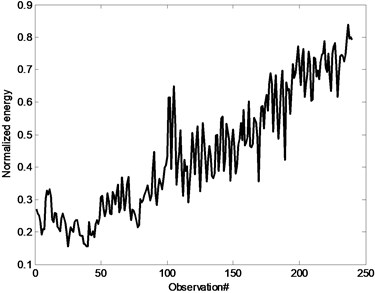The prediction analysis based on the 150th and 200th points acquisition data are carried on, shown as Fig. 11 and Fig. 12 based on SSM. For the 150th point, the mean value based on the prediction deviates from the degradation trend. The confidence interval can't reflect the prediction accuracy. Although the mean value is not the same with the degradation process for 200th point prediction, it is almost same with the degradation process. There is also difference for the confidence interval. It is obviously the analysis result is not good for the prediction. It is important to improve the prediction accuracy. It also means that SSM depends on the degradation process. The result has been greatly influenced by the data fluctuation shown as Fig. 11 and Fig. 12. Prediction accuracy is influenced as the data fluctuation. Therefore, it is important to process the data for accurate analysis. To improve the prediction accuracy, moving average is carried on for the analysis.

Fig. 11Prediction data for the 150th step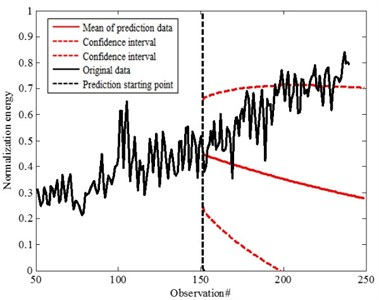Fig. 12Prediction data for the 200th step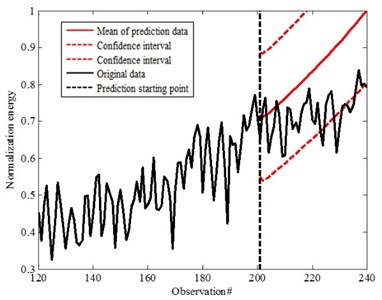### 4.2. Moving average

Moving averageis a data pre-processing method with smoothing and filtering effect. It can filter out the frequent stochastic fluctuant data . At the same time, it can also conclude the changing process of random error. Thus, the statistical characteristics can be estimated. As shown in Fig. 13, let the length $L$ sliding window move through the sequence $\left\{y\left(t\right),t=1,2,\dots ,N\right\}$. Then, compute the mathematic average for all elements inside the window and put the average value as one element of the new sequence, and the new sequence is moving average of $y\left(t\right)$. Mathematical expression is as follows:

38
${y}_{ma}\left(t\right)=\frac{1}{L}\sum _{t=L+i}^{2L+i-1}y\left(t\right),i=0,1,2,\dots ,N-2L+1.$

Fig. 13The moving average method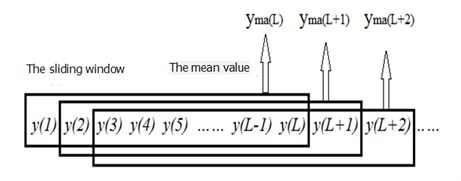Based on the moving average process, taking 50 observed values for average. Fig. 14 presents the comparison for original one and after moving average. It is obvious that the degradation data trend is almost linear after moving average. It can effectively reduce the data fluctuation for the effect of prediction. It is good to improve the accuracy for prediction. As it is almost linear, Kalman filter is better for the SSM prediction. Initial parameters setting is as following for the SSM prediction:

39
$A=\left[\begin{array}{cc}1& 0\\ 0& 1\end{array}\right],Q=\left[\begin{array}{cc}1& 0\\ 0& 1\end{array}\right],C=\left[\begin{array}{c}0\\ 1\end{array}\right],{\mu }_{0}=\left[\begin{array}{c}0\\ 0\end{array}\right],{\epsilon }_{0}=\left[\begin{array}{cc}1& 1\\ 0& 0\end{array}\right],R=\left[1\right].$

Fig. 14Comparison for original data and after moving average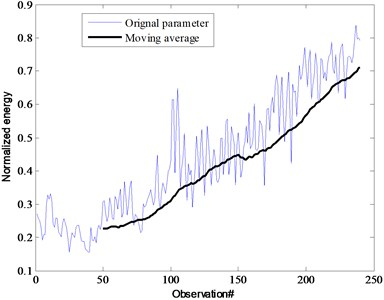Fig. 15 and Fig. 16 are the prediction results of SSM established by observed values of two different time steps 150th and 200th point based on different confidence 90 % and 95 %. The mean of prediction for the 200th point is almost the same with original data (characteristic parameters after moving average) which is within the confidence interval. In this research, the confidence is defined as 90 %. It is better compared with 150th point prediction. It is also obvious that the prediction accuracy can be improved with time step increment based on comparison for different working conditions. But it is much better compared with Fig. 11 and Fig. 12 for the data without moving average. That also means it could effectively improve the accuracy with moving average. Data preprocessing is important to assure the accuracy prediction. By using moving average, the fluctuation from the characteristic parameters can be effectively removed. It is also helpful to improve the prediction accuracy. As well, confidence also has effect on the analysis. Based on the prediction, the development is within the confidence.

Fig. 17 is the reliability prediction results of the corresponding MC. The actual life of cutter is 236. Set the reliability threshold $R=$ 0.5. The prediction nonfatal failure moment distribution of cutter is 229 and 235, which is consistent with the actual situation. It is better for 150th though it is a little far from the failure point. But it can also present accurate prediction result. It is much better for 200th prediction compared with 150th. As well, it can significantly reduce the prediction error and improve the prediction accuracy. Based on the parameters updated for every step prediction, it can be obtained that the prediction will get more and more accurate with parameters update. Therefore, this method is effectively verified to the analysis of practical problems. It can be used on the prediction and reliability analysis for MC wearing condition as this is real working machine.

Fig. 15Prediction data of 90 % confidence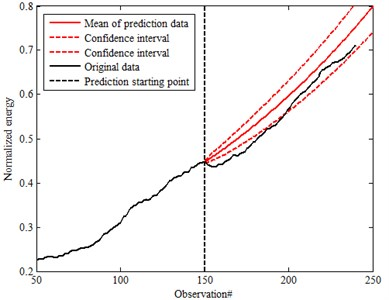a) The 150th prediction for moving average data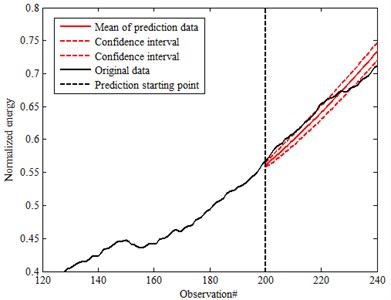b) The 200th prediction for moving average data

Fig. 16Prediction data of 95 % confidence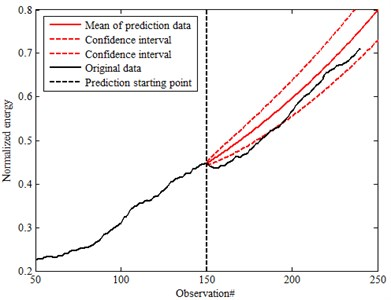a) The 150th prediction for moving average data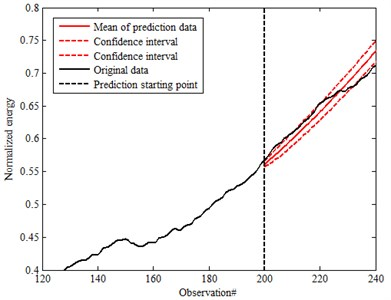b) The 200th prediction for moving average data

Fig. 17The reliability prediction results at different time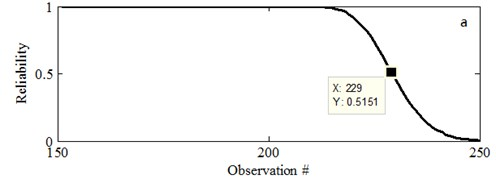a) Prediction based on 150#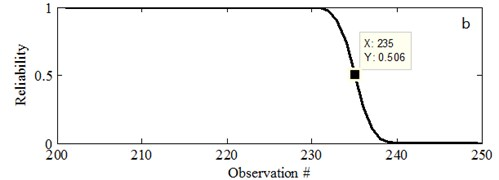b) Prediction based on 200#

### 4.3. Discussions

Based on above analysis, it is clear the SSM has good performance on prediction analysis as it is based on EM process. It is an iteration process which is just based on the current data for prediction. Therefore, it is good for reliability analysis. At the same time, the prediction result is also influenced by the data fluctuation if there is not moving average process. It also demonstrates the degradation trend which is very important for the prediction and reliability analysis. How to effectively determine the best suitable parameters for condition reliability analysis is the basis.

AE signal is good to demonstrate the wearing process for MC and failure process. It is good for moving average process to reduce fluctuation effect for SSM analysis based on the feature extraction. Actually, only a parameter is used in this investigation to verify the effectiveness of SSM model on prediction. Condition monitoring is the dispersive for different data. At the same time, it needs more information to estimate machine working conditions and reliability analysis. It is better to use much data analysis for reliability analysis. But it depends on the monitoring process and the degradation process for different parameters. The monitored data can be integrated together for better demonstration of machine condition parameters. Then, a parameter is used for reliability analysis. Otherwise, more monitored parameters can be input in Eq. (9). The prediction process can be carried on for analysis. As the degradation process is not linear for more parameters prediction, improved Kalman filter can be used for analysis.

## 5. Conclusions

In this research, a new method for milling cutter reliability estimation method is put forward based on feature extraction and SSM. Wavelet packet feature extraction, moving average, and SSM are combined together for condition estimation and reliability analysis. Based on the experimental analysis, the effectiveness of this method is demonstrated. The suitable parameter is important for reliability analysis. As well, accuracy feature extraction is beneficial to determine the degradation trend. The result shows that the moving average filtering can effectively improve the signal noise ratio of the degradation characteristics and improve the accuracy of the prediction. It is accorded with the time dynamic characteristics of the equipment performance degradation. This method has good performance on reliability analysis. Further investigation should be carried on the application of this method on practical question analysis.

#### Cited by

18 November 2015
Accepted
05 September 2016
Published
15 November 2016
SUBJECTS
Fault diagnosis based on vibration signal analysis
Keywords
reliability prediction
state space model
feature extraction
moving average
milling cutter
Acknowledgements

The support from Chinese National Science Foundation (Grant No. 51575075 and 51175057).